### Home > CALC > Chapter 12 > Lesson 12.2.2 > Problem12-79

12-79.
1. Consider the infinite series below. For each, decide if it converges or diverges and justify your conclusion. State which test you used. Homework Help ✎

1.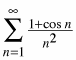2.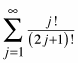3.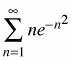4.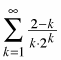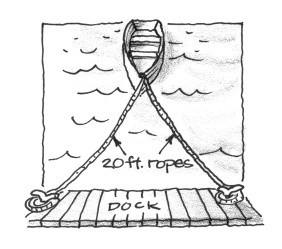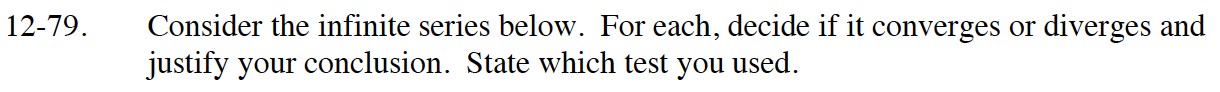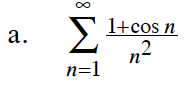$\text{Compare this series to }\sum_{n=1}^\infty \frac{2}{n^2}.$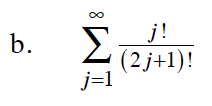$\lim_{j\to\infty}\Bigg|\frac{\frac{(j+1)!}{(2j+2)!}}{\frac{j!}{(2j+1)!}}\Bigg|$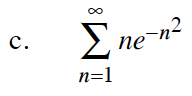$\lim_{n\to\infty}\Bigg|\frac{\frac{n+1}{e^{(n+1)^2}}}{\frac{n}{e^{n^2}}}\Bigg|$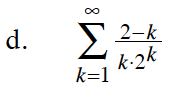$\lim_{k\to\infty}\Bigg|\frac{\frac{1-k}{(k+1)\cdot 2^{k+1}}}{\frac{2-k}{k\cdot 2^k}}\Bigg|$